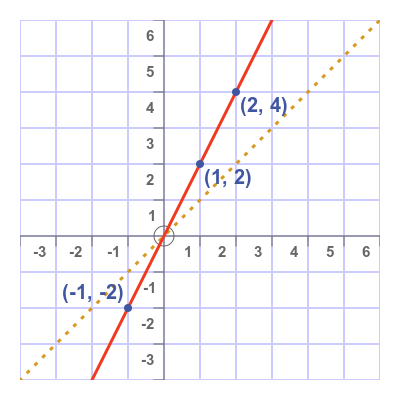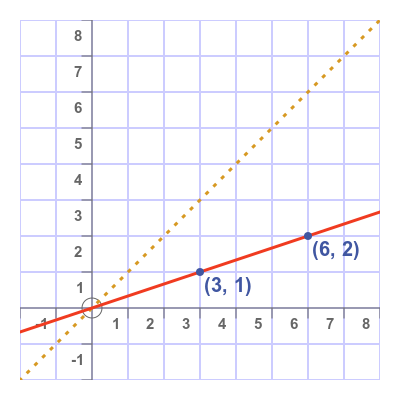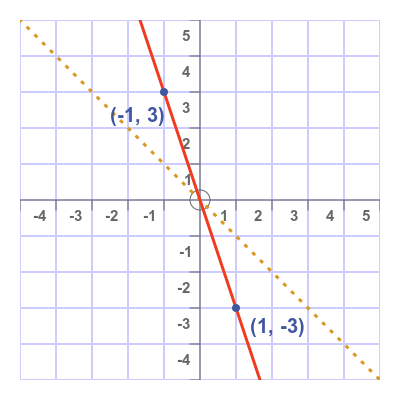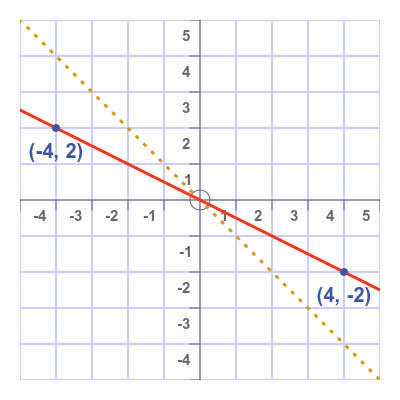# Graphs of sloping lines through the origin

Martin McBride
2022-05-25

In this section, we will look at the graphs of sloping straight lines that go through the origin.

These lines all have the equation:

$$y = a x$$

but use different values for a.

## The line y = 2x

This graph shows a graph of the straight line y = 2x:We can calculate points that are on the line, using the formula. We simply chose an x-value (for example 2), and multiply it by 2 to get the y-value (which would be 4). Here are some other points that are marked on the graph:

• (-1, -2)
• (1, 2)
• (2, 4)

The dashed yellow line has the equation y = x. We can see that the line y = 2x is steeper than the line y = x.

## The line y = ⅓x

This graph shows a graph of the straight line y = ⅓x:Again, we can calculate points that are on the line. We simply chose an x-value (for example 3), and multiply it by one third.

Multiplying by one third is the same as dividing by 3, so this would give a y-value of 1. Here are some other points that are marked on the graph:

• (3, 1)
• (6, 2)

We can see that this time the line is less steep than the line y = x.

## Using negative values

We can use a negative multiplier, for example, y = -3x. In this case, if we take an x-value of 1, we get a y-value of -3. Similarly, for an x-value of -1, we get a y-value of 3.

Here is the graph:This line slopes downwards. Compared to the dashed yellow line y = -x, the line y = -3x is steeper but in the negative direction.

Here is a graph of the equation y = -½x:This graph goes through the points (-4. 2) and (4, -2), calculated in the same way as before. This time the curve is less steep than y = -x.

## Rules

• A straight line passing through the origin has the equation y = ax.
• If a is positive the line slopes upwards.
• If a is negative the line slopes downwards.
• The greater the absolute value of a, the steeper the slope.